# Home

 Pauli's Spin Matrices 7th September 2015 The space-time position (c2t2 - x2- y2- z2), an invariant under Lorentz transformation, is given by the (ct, x, y, z) metric in Clifford Algebra as,If the observer has an infinite capacity we can reduce the problem to the single measurement or a pure two-state system.  The single measurement capacity implies relativistic limits i.e. zero entropy ⇒ t ≈ 0*.  In that case matrix X reduces to zero-entropy space-time matrix Xo,Writing Xo into its components, we get the following matrices: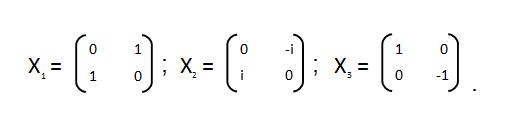X1, X2, and X3 are known as Pauli Spin Matrices.  The matrix X3 corresponds to the actual physical measurement of a two state system with eigenvalues (EVs) [1, -1] or the measurements along z-axis.  The off-diagonal elements equal to '0' represent zero probability of tunneling between the states represented by EVs 1 and -1.  Pauli matrices are conventionally represented as σx ,  σy ,  and σz.     An important property to note is that Pauli Spin Matrices are their own inverse(s), which means that the definition of the origin is mandatory within the structure described by these matrices.  (It is not necessarily true for a simple reflection of a structure in which case the reference origin is in the plane of the mirror, not in that of the structure itself).  As we recall that the VT symmetry is required to determine the origin, hence the quanta for the measurement space is pre-defined for the structure described by these matrices.  Please also note that Pauli spin matrices do not represent Majorana states.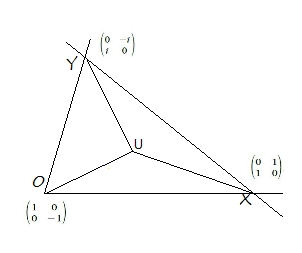When we see a structure like the measurement triangle ΔOXY in anharmonic coordinates, Pauli's spin matrices come to mind immediately.  Let us try to evaluate the measurement triangle ΔOXY by placing σx , σy , and σz at X, Y, and O respectively and measure the unit point U.  Effectively we have replaced the α, β, and γ by spin matrices, and moved the system into a two-component measurement space.  We should be able to find scalars l, m and n such that,                                          lσx + mσy + nσz = 0 .               -1 We plug in the values of matrices and solve to find,                                        l2 + m2 + n2 = 0 .                    -2 The equation-2 represents an sphere in measurement space with radius zero as measured by the macroscopic observer ObsM.  Please note that l, m, n, are the points lying on the measurement net.  Each of them is an integer or a ratio of integers hence rational or "constructible"**.  This is how the most elementary geometrical symmetry i.e. the spherical symmetry, is formed from a two-state system.     The unit-point U will be measured as an sphere of zero radius by ObsM.  Let us call it unit-point sphere SU***.  This situation is rather fantastic.  The quantities on LHS of equation-2 are positive, whereas RHS is equal to zero.  However we must not forget Obsi, the i-space observer who has higher capacity than ObsM.  Obsi will measure 0 as 0j, a finite value.  Hence equation-2 can be rewritten as,                                                               l2 + m2 + n2 = 0j .                      -3   Above equation represents a sphere of finite radius √0j, a radius whose measurement is not possible for ObsM.  We should be using the quantity i√0j or an imaginary value for radius, but we are not.  Why?  Because in measurement space only PE1 measurable quantities are reported, hence only 0j which actually is the radius squared, is of value.   In other words the information on the internal structure of this sphere is not available to the macroscopic observer ObsM.  In a later section, this argument will result in shell-structure we are so familiar in classical picture.      Interestingly enough, we can replace Pauli's spin matrices with the components of Quaternion matrix in equation-1.  In this case also the equation-2 and the equation-3 remain unchanged.  Therefore in both cases, in which the component matrices represent convex surfaces, the geometric net results in spheres of finite radii.  Both these spheres represent stable structures.  One just wonders, what type of current will result from such symmetry?  It should be noted that the value of 0j will be different in each case.     The unit-point sphere SU has a symmetry of 4π or 720o, centered around the unit point U.  The 4π-symmetry is an essential feature of the systems with interacting components, or the systems whose components are not completely independent of each other.  We can later build a coordinate system with independent axes with SU with 4π- symmetry, as the origin.  We can visualize the symmetry of 4π as a fundamental internal symmetry of a two-state system, measured as SU by ObsM at Λ∞.     One may want to think of the symmetry with respect to the integral multiple or half- integral multiple rotation of 360o, but then not all the information contained within SU would be measured.  Had we considered only a classical single-state system, the 360o rotation would have been sufficient to describe the corresponding symmetry.  Obviously, two 360o rotations do not represent 4π-symmetry which is the symmetry of the origin itself.  In fact the information measured by ∞j × 360o rotations, will always be less than the information contained within the 4π-symmetry.  In other words the resources available to a classical observer are not enough to re-define the origin and change the information structure being measured.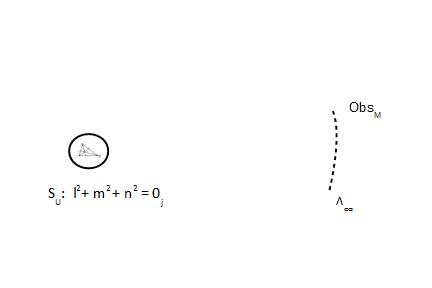The unit-line Λ∞ has the property, that it is parallel to every straight line in the anharmonic plane.  We have used this property for drawing an important geometric physical feature related to ObsM.  Can you figure out this feature?  Think shell theorems.       Please note that SU will form the initial state for the measurements performed by ObsM, the measurements represented by the polar Λ∞ in anharmonic coordinates.  The central force nature of the problem is quite evident.  We have so far refrained from using physical constants or known forces in nature while developing this description.  One of the conditions we have discussed, is related to the values of scalars l, m, and n, which have to be either integer or rational.  Consequently a continuous spectrum of measurable structures is prohibited in j-space.  In other words a continuous spectrum for properties similar to mass can not be measured in j-space.  In fact in pure j-space, continuous spectrum corresponding to any property related to a stable structure, can not be measured. __________________________________________________________   *The complete Hamiltonian of a two-state system is given as,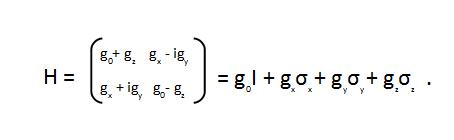We have merely eliminated the time-dependent phase which is a function of g0 to derive a description with the unit point U as reference.  Please note that we have thus introduced the relativistic description by making time insignificant.  For the unit point U the time has no significance, as time is the property associated with the observer making measurement, not with the source which is being measured.  The conventional (t, x, y, z) description is valid only for Λ∞, the measurement plane of the macroscopic observer with intrinsic entropy. ** What happens if  (l, m, n) are complex numbers? *** The unit-point sphere SU is not the same as traditional unit sphere S 2.Previous Blogs: Sigma-z and I Spin Matrices Rational behind Irrational Numbers The Ubiquitous z-Axis Majorana ZFC Axioms Set Theory Nutshell-2014 Knots in j-Space Supercolliders Force Riemann Hypothesis Andromeda Nebula Infinite Fulcrum Cauchy and Gaussian Distributions Discrete Space, b-Field & Lower Mass Bound Incompleteness II The Supersymmetry The Cat in Box The Initial State and Symmetries Incompleteness I Discrete Measurement Space The Frog in Well Visual Complex Analysis The Einstein Theory of Relativity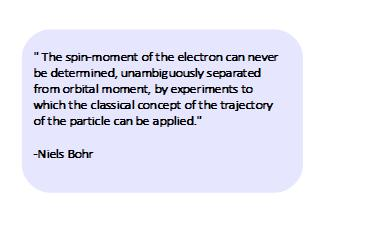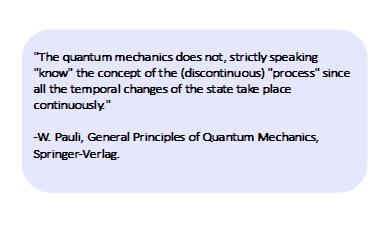Best viewed with Internet Explorer